# GPy Introduction: Covariance Functions in GPy¶

## Gaussian Process Winter School, Genova, Italy¶

### Neil D. Lawrence and Nicolas Durrande¶

In :
%matplotlib inline
from IPython.display import display
import numpy as np
import matplotlib.pyplot as plt
import pods
import GPy

/Users/neil/Library/Enthought/Canopy_64bit/User/lib/python2.7/site-packages/pytz/__init__.py:29: UserWarning: Module pods was already imported from /Users/neil/sods/ods/pods/__init__.pyc, but /Users/neil/Library/Enthought/Canopy_64bit/User/lib/python2.7/site-packages is being added to sys.path
from pkg_resources import resource_stream


## Covariance Functions in GPy¶

We've introduced Gaussian processes and built a simple class in python for fitting these models. In the last session we introduced Gaussian process models through constructing covariance functions in numpy. The GPy software is a BSD licensed software package for modeling with Gaussian processes in python. It is designed to make it easy for the user to construct models and interact with data. The software is BSD licensed to ensure that there are as few as possible constraints on its use. The GPy documentation is produced with Sphinx and is available here.

In the introduction to Gaussian processes we defined covariance functions (or kernels) as functions to which we passed objects in GPy covariance functions are objects.

In GPy the covariance object is stored in GPy.kern. There are several covariance functions available. The exponentiated quadratic covariance is stored as RBF and can be created as follows.

In :
kern = GPy.kern.RBF(input_dim=1)
display(kern)

rbf. Value Constraint Prior Tied to
variance 1.0 +ve
lengthscale 1.0 +ve

Here it's been given the name 'rbf' by default and some default values have been given for the lengthscale and variance, we can also name the covariance and change the initial parameters as follows,

In :
kern = GPy.kern.RBF(input_dim=1, name='signal', variance=4.0, lengthscale=2.0)
display(kern)

signal. Value Constraint Prior Tied to
variance 4.0 +ve
lengthscale 2.0 +ve

### Plotting the Kernel¶

The covariance function expresses the covariation between two points. the .plot() method can be applied to the kernel to discover how the covariation changes as a function as one of the inputs with the other fixed (i.e. it plots k(x, z) as a function of a one dimensional x whilst keeping z fixed. By default z is set to 0.0.

In :
kern.plot()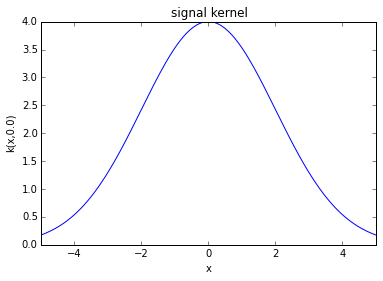Here the title of the kernel is taken from the name we gave it (signal).

## Changing Covariance Function Parameters¶

When we constructed the covariance function we gave it particular parameters. These parameters can be changed later in a couple of different ways firstly, we can simple set the field value of the parameters. If we want to change the lengthscale of the covariance to 3.5 we do so as follows.

In :
kern.lengthscale = 3.5
display(kern)

signal. Value Constraint Prior Tied to
variance 4.0 +ve
lengthscale 3.5 +ve

We can even change the naming of the parameters, let's imagine that this covariance function was operating over time instead of space, we might prefer the name timescale for the lengthscale parameter.

In :
kern.lengthscale.name = 'timescale'
display(kern)

signal. Value Constraint Prior Tied to
variance 4.0 +ve
timescale 3.5 +ve

Now we can set the time scale appropriately.

In :
kern.timescale = 10.
display(kern)

signal. Value Constraint Prior Tied to
variance 4.0 +ve
timescale 10.0 +ve

## Further Covariance Functions in GPy¶

There are other types of basic covariance function in GPy. For example the Linear kernel, $$k(\mathbf{x}, \mathbf{z}) = \alpha \mathbf{x}^\top \mathbf{z}$$ and the Bias kernel, $$k(\mathbf{x}, \mathbf{z}) = \alpha$$ where everything is equally correlated to each other. Brownian implements Brownian motion which has a covariance function of the form, $$k(t, t^\prime) = \alpha \text{min}(t, t^\prime).$$

Broadly speaking covariances fall into two classes, stationary covariance functions, for which the kernel can always be written in the form $$k(\mathbf{x}, \mathbf{z}) = f(r)$$ where $f(\cdot)$ is a function and $r$ is the distance between the vectors $\mathbf{x}$ and $\mathbf{z}$, i.e., $$r = ||\mathbf{x} - \mathbf{z}||_2 = \sqrt{\left(\mathbf{x} - \mathbf{z}\right)^\top \left(\mathbf{x} - \mathbf{z}\right)}.$$ This partitioning is reflected in the object hierarchy in GPy. There is a base object Kern and this is inherited by Stationary to form the stationary covariance functions (like RBF and Matern32).

## Computing the Covariance Function¶

When using numpy to construct covariances we defined a function, kern_compute which returned a covariance matrix given the name of the covariance function. In GPy, the base object Kern implements a method K. That allows us to compute the associated covariance. Visualizing the structure of this covariance matrix is often informative in understanding whether we have appropriately captured the nature of the relationship between our variables in our covariance function. In GPy the input data is assumed to be provided in a matrix with $n$ rows and $p$ columns where $n$ is the number of data points and $p$ is the number of features we are dealing with. We can compute the entries to the covariance matrix for a given set of inputs X as follows.

In :
data = pods.datasets.olympic_marathon_men()
# Load in the times of the olympics
X = data['X']
K=kern.K(X)


Now to visualize this covariation between the time points we plot it as an image using the imshow command from matplotlib.

plt.imshow(K, interpolation='None')


Setting the interpolation to 'None' prevents the pixels being smoothed in the image. To better visualize the structure of the covariance we've also drawn white lines at the points where the First World War and the Second World War begin. At other points the Olympics are held every four years and the covariation is given by the computing the covariance function for two points which are four years apart, appropriately scaled by the time scale.

In :
def visualize_olympics(K):
"""Helper function for visualizing a covariance computed on the Olympics training data."""
fig, ax = plt.subplots(figsize=(8,8))
im = ax.imshow(K, interpolation='None')

WWI_index = np.argwhere(X==1912)
WWII_index = np.argwhere(X==1936)

ax.axhline(WWI_index+0.5,color='w')
ax.axvline(WWI_index+0.5,color='w')
ax.axhline(WWII_index+0.5,color='w')
ax.axvline(WWII_index+0.5,color='w')
plt.colorbar(im)

visualize_olympics(kern.K(X))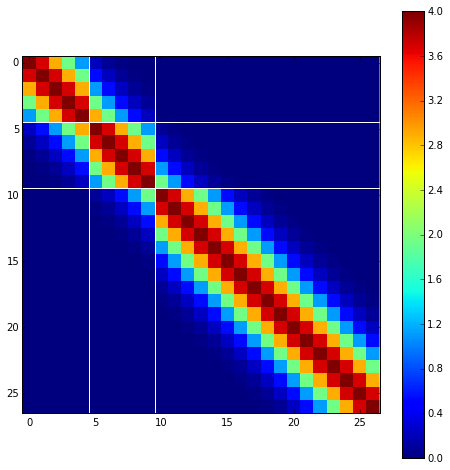In :
kern.timescale

Out:
Index signal.timescale Constraint Prior Tied to
10.0+veN/A

of 10 is ensuring that neighbouring Olympic years are correlated, but there is only weak dependency across the period of each of the world wars. If we increase the timescale to 20 we obtain stronger dependencies between the wars.

In :
kern.timescale = 20
visualize_olympics(kern.K(X))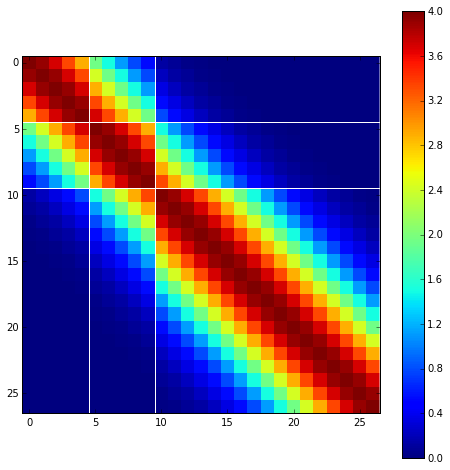## Covariance Matrices and Covariance Functions¶

A Gaussian process specifieds that any finite set of realizations of the function will jointly have a Gaussian density. In other words, if we are interested in the joint distribution of function values at a set of particular points, realizations of those functions can be sampled according to a Gaussian density. The Gaussian process provides a prior over an infinite dimensional object: the function. For our purposes we can think of a function as being like an infinite dimensional vector. The mean of our Gaussian process is normally specified by a vector, but is now itself specified by a mean function. The covariance of the process, instead of being a matrix is also a covariance function. But to construct the infinite dimensional matrix we need to make it a function of two arguments, $k(\mathbf{x}, \mathbf{z})$. When we compute the covariance matrix using k.K(X) we are computing the values of that matrix for the different entries in $\mathbf{X}$. GPy also allows us to compute the cross covariance between two input matrices, $\mathbf{X}$ and $\mathbf{Z}$.

## Sampling from a Gaussian Process¶

We cannot sample a full function from a process because it consists of infinite values, however, we can obtain a finite sample from a function as described by a Gaussian process and visualize these samples as functions. This is a useful exercise as it allows us to visualize the type of functions that fall within the support of our Gaussian process prior. Careful selection of the right covariance function can improve the performance of a Gaussian process based model in practice because the covariance function allows us to bring domain knowledge to bear on the problem. If the input domain is low dimensional, then we can at least ensure that we are encoding something reasonable in the covariance through visualizing samples from the Gaussian process.

For a one dimensional function, if we select a vector of input values, represented by X, to be equally spaced, and ensure that the spacing is small relative to the lengthscale of our process, then we can visualize a sample from the function by sampling from the Gaussian (or a multivariate normal) with the numpy command

F = np.random.multivariate_normal(mu, K, num_samps).T


where mu is the mean (which we will set to be the zero vector) and K is the covariance matrix computed at the points where we wish to visualize the function. The transpose at the end ensures that the the matrix F has num_samps columns and $n$ rows, where $n$ is the dimensionality of the square covariance matrix K.

Below we build a simple helper function for sampling from a Gaussian process and visualizing the result.

In :
def sample_covariance(kern, X, num_samps=10):
"""Sample a one dimensional function as if its from a Gaussian process with the given covariance function."""
from IPython.display import HTML
display(HTML('<h2>Samples from a Gaussian Process with ' + kern.name + ' Covariance</h2>'))
display(kern)
K = kern.K(X)

# Generate samples paths from a Gaussian with zero mean and covariance K
F = np.random.multivariate_normal(np.zeros(X.shape), K, num_samps).T

fig, ax = plt.subplots(figsize=(8,8))
ax.plot(X,F)


We are now in a position to define a vector of inputs, a covariance function, and then to visualize the samples from the process.

In :
# create an input vector
X = np.linspace(-2, 2, 200)[:, None]

# create a covariance to visualize
kern = GPy.kern.RBF(input_dim=1)

# perform the samples.
sample_covariance(kern, X)


## Samples from a Gaussian Process with rbf Covariance

rbf. Value Constraint Prior Tied to
variance 1.0 +ve
lengthscale 1.0 +ve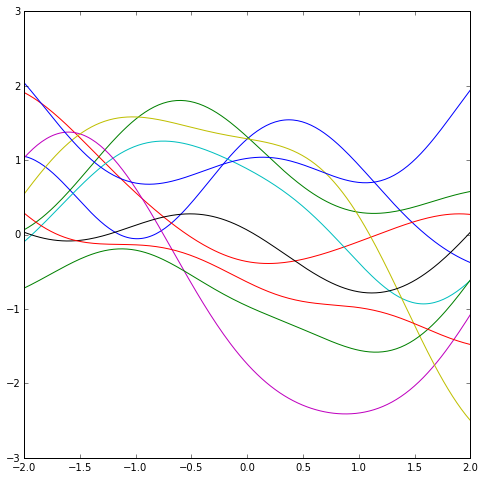In :
kern = GPy.kern.Poly(input_dim=1, order=6)
X = np.linspace(-.8, .8, 500)[:, None]
sample_covariance(kern, X)


## Samples from a Gaussian Process with poly Covariance

poly. Value Constraint Prior Tied to
variance 1.0 +ve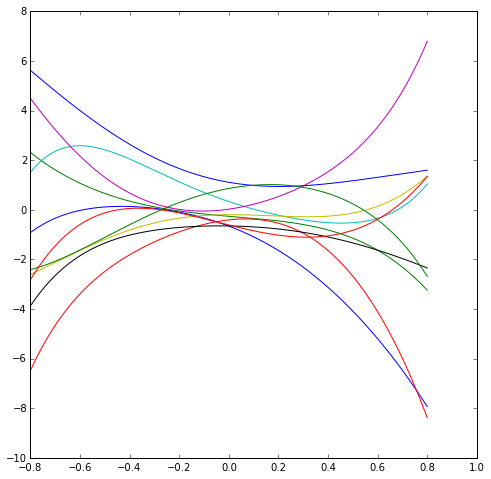## Combining Covariance Functions¶

Covariance functions can be combined in various ways to make new covariance functions.

Perhaps simplest thing you can do to combine covariance functions is to add them. The sum of two Gaussian random variables is also Gaussian distributed, with a covariance equal to the sum of the covariances of the original variables (similarly for the mean). This implies that if we add two functions drawn from Gaussian processes together, then the result is another function drawn from a Gaussian process, and the covariance function is the sum of the two covariance functions of the original process. $$k(\mathbf{x}, \mathbf{z}) = k_1(\mathbf{x}, \mathbf{z}) + k_2(\mathbf{x}, \mathbf{z}).$$ Here the domains of the two processes don't even need to be the same, so one of the covariance functions could perhaps depend on time and the other on space, $$k\left(\left[\mathbf{x}\quad t\right], \left[\mathbf{z}\quad t^\prime\right]\right) = k_1(\mathbf{x}, \mathbf{z}) + k_2(t, t^\prime).$$

In GPy the addition operator is overloaded so that it is easy to construct new covariance functions by adding other covariance functions together.

In :
k1 = GPy.kern.RBF(1, variance=4.0, lengthscale=10., name='long term trend')
k2 = GPy.kern.RBF(1, variance=1.0, lengthscale=2., name='short term trend')
kern = k1 + k2
kern.name = 'signal'
kern.long_term_trend.lengthscale.name = 'timescale'
kern.short_term_trend.lengthscale.name = 'timescale'
display(kern)

signal. Value Constraint Prior Tied to
long term trend.variance 4.0 +ve
long term trend.timescale 10.0 +ve
short term trend.variance 1.0 +ve
short term trend.timescale 2.0 +ve

### Multiplying Covariance Functions¶

An alternative is to multiply covariance functions together. This also leads to a valid covariance function (i.e. the kernel is within the space of Mercer kernels), although the interpretation isn't as straightforward as the additive covariance.

In :
k1 = GPy.kern.Linear(1)
k2 = GPy.kern.RBF(1)
kern = k1*k2
display(kern)
fig, ax = plt.subplots(figsize=(8,8))
im = ax.imshow(kern.K(X), interpolation='None')
plt.colorbar(im)

mul. Value Constraint Prior Tied to
linear.variances 1.0 +ve
rbf.variance 1.0 +ve
rbf.lengthscale 1.0 +ve
Out:
<matplotlib.colorbar.Colorbar instance at 0x10f846710>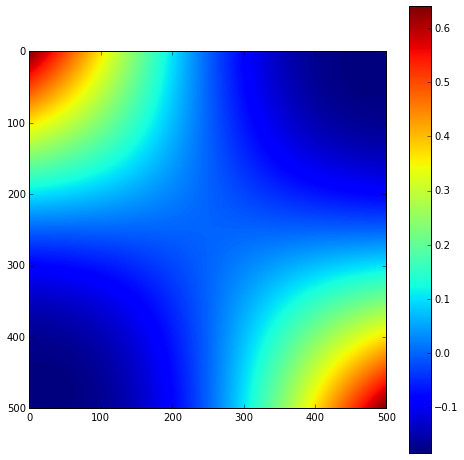### Exercise 3¶

Now try a range of different covariance functions and values and plot the corresponding sample paths for each using the same approach given above.

In :
# Try plotting sample paths here


### Exercise 4¶

Can you tell the covariance structures that have been used for generating the sample paths shown in the figures below?